Courses

# Additional Questions Solutions- Constructions Class 9 Notes | EduRev

## Mathematics (Maths) Class 9

Created by: Full Circle

## Class 9 : Additional Questions Solutions- Constructions Class 9 Notes | EduRev

The document Additional Questions Solutions- Constructions Class 9 Notes | EduRev is a part of the Class 9 Course Mathematics (Maths) Class 9.
All you need of Class 9 at this link: Class 9

Question 1. Construct a triangle ABC in which BC = 7.5 cm, ∠ B = 45º and the difference between the other two sides is 4 cm.
Solution: Steps of construction:

I . Take BC = 7.5 cm.
II. Construct ∠CBX = 45º.
III. From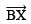cut off BD = 4 cm.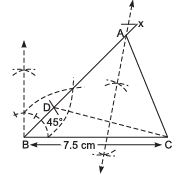IV. Join D and C.
V. Draw the perpendicular bisector of DC which intersectsat A.
VI. Join A and C.
Thus, ABC is the required triangle.

Question 2. Construct a ΔABC whose perimeter is 12 cm and sides are in the ratio 2 : 3 : 4.
Solution: Steps of construction: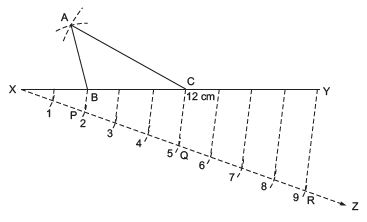I . Draw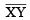= 12 cm.
II. Draw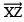, making an acute angle with XY in the downward direction.
III. From X, set off (2 + 3 + 4 = 9) nine equal distances along XZ.
IV. Mark points P, Q and R on XZ such that XP = 2 units PQ = 3 units QR = 4 units
V. Join RY.
VI. Draw QC || RY and PB || RY.
VII. With centre B and radius BX, draw an arc.
VIII. With centre C and radius CY, draw an arc which intersects previous arc at A.
IX. Join AB and AC.
Thus, ΔABC is the required triangle.

Question 3. Construct ΔABC, in which ∠ B = 60º and ∠ C = 45º and the perpendicular from the vertex A to the base BC is 5.2 cm.
Solution: Steps of construction: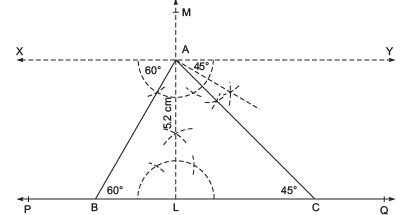I . Draw a line PQ.
II . Mark a point L on PQ.
III. Draw LM ⊥ PQ.
IV. From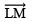cut off LA = 5.2 cm.
V. Draw XY || PQ, through A.
VI. Construct ∠XAB = 60º and ∠YAC = 45º meeting PQ at B and C respectively.
Thus, ΔABC is the required triangle.

Question 4. Contruct an equilateral triangle if its altitude is 6 cm. Give justification for your construction.
Solution: Steps of construction: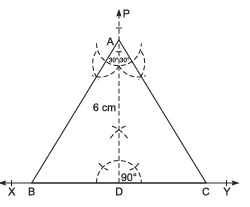I . Draw a line XY and mark a point D on it.
II. Construct perpendicular PD on XY.
III. Cut off AD = 6 cm
IV. Make ∠CAD = 30° and ∠BAD = 30° at A on both sides of AD, such that B and C lie on XY.
Thus, ABC is the required triangle.
Justification:
∴ In ΔADC, ∠C = 180 ∠ (90° + 30°) = 60°
⇒ ∠A = 60°
Thus, ΔABC is an equilateral triangle in which altitude AD = 6 cm.

## Mathematics (Maths) Class 9

192 videos|230 docs|82 tests

,

,

,

,

,

,

,

,

,

,

,

,

,

,

,

,

,

,

,

,

,

;